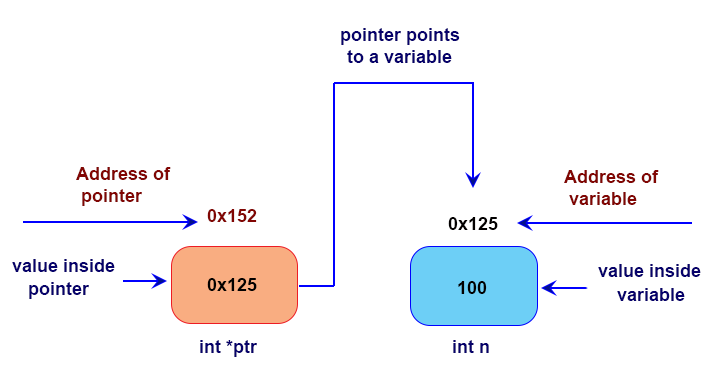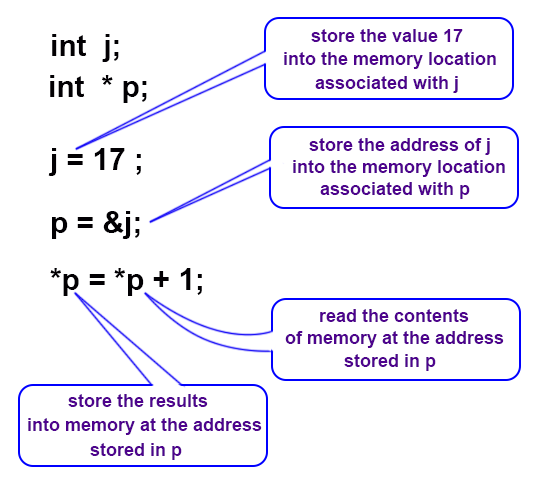# C - Pointers

## What is a Pointer?

A pointer is a variable that stores the address of a memory location. Pointers are used to store the addresses of other variables or memory items. A pointer is extremely helpful for another type of parameter passing, commonly referred to as pass by address. Pointers are essential for dynamic memory allocation.The following are some common uses for pointers:

• To access dynamic data structures such as linked lists, trees, and queues.
• To access elements of an array or members of a structure.
• To access an array of characters as a string.
• To pass the address of a variable to a function.

Declaring a pointer variable

Pointer declarations use the * operator. They follow this format:

• int n; // declaration of a variable n
• int * ptr; // declaration of a pointer, called p

In the example above, ptr is a pointer, and its type will be specifically be referred to as "pointer to int", because it stores the address of an integer variable. We also can say its type is: int*

The type is important. While pointers are all the same size, as they just store a memory address, we have to know what kind of thing they are pointing to.

• double * dptr; // a pointer to a double
• char * ch; // a pointer to a character
• float * fptr; // a pointer to a float

Sometimes the notation is confusing, because different textbooks place the * differently. The three following declarations are equivalent:

• int *ptr;
• int* ptr;
• int * ptr;

Above three of these declare the variable ptr as a pointer to an int.

Another tricky aspect of notation: Recall that we can declare multiple variables on one line under the same type, like this:

• int x, y, z; // three variables of type int

Since the type of a "pointer-to-int" is (int *), we might ask, does this create three pointers?

• int* a, b, c;

This is not three pointers. Instead, this is one pointer and two integers. If you want to create multiple pointers on one declaration, you must repeat the * operator each time:

• int * a, * b, * c; // three pointers-to-int
• int * a, b, c; // a is a pointer, b and c are integers.

Assigning to pointer variables

A variable with a pointer value allocates space to hold the pointer, but not to hold anything it points to. As with any other variable in C, a pointer-valued variable will initially contain garbage - in this case, the address of a location which may or may not contain some useful information. A pointer variable is initialized by assigning it the address of something that already exists. The & (address-of) operator is commonly used to accomplish this:

• int x; /* an int variable */
• int *ptr; /* a pointer to an int */
• ptr = &x; /* p now points to n */

Operators

• *ptr -- returns the value pointed to by ptr
• &x -- returns the address of variable x

Using a pointer

There are two ways to use pointer variables:

a) To get their value (a pointer) –

• int x; /* an int variable */
• int *a; /* a pointer to an int */
• int *b; /* another pointer to an int */
• a = &x; /* a now points to x */
• b = a; /* b now points to x as well */

b) In most cases, however, you will want to work with the value stored at the location indicated. You can do this by using the * (dereference) operator:

• int x; /* an int variable */
• int *a; /* a pointer to an int */
• a = &x; /* a now points to x */
• *a = 2; /* a now points to x */
• *a = *a + *a; /* sets n to 4 */

Example:A pointer in C is always a pointer to a particular data type:
int*, double*, char*, etc.

Integer pointer:

An integer pointer stores only the address of an integer variable.

Syntax:

```int *p;    // *p is the name of pointer
```

Example: Integer pointer

Code:

``````#include<stdio.h>
int main()
{
int x = 25;
int *p;
p = &x;
printf("Value of x is: %d\n",x);
printf("Value stored at pointer p is: %d\n",*p);
printf("Address of the variable x is: %x\n",&x);
printf("p points to the address = %x\n",p);
printf("Address of the pointer p = %x\n",&p);
return 0;
}
``````

Output:

```Value of x is: 25
Value stored at pointer p is: 25
Address of the variable x is: 62fe1c
p points to the address = 62fe1c
Address of the pointer p = 62fe10
```

Character pointer:

A character pointer stores only the address of a character variable.

Syntax:

```char *p; // *p is the name of pointer
```

Example: Charcter pointer

Code:

``````#include<stdio.h>
int main()
{
char ch = 'W',*p;
p = &ch;
printf("Value of ch is: %c\n",ch);
printf("Value stored at pointer p is: %c\n",*p);
printf("Address of the variable ch is: %x\n",&ch);
printf("p points to the address = %x\n",p);
printf("Address of the pointer p = %x\n",&p);
return 0;
}
``````

Output:

```Value of ch is: W
Value stored at pointer p is: W
Address of the variable ch is: 62fe1f
p points to the address = 62fe1f
Address of the pointer p = 62fe10
```

Floating pointer:

An integer pointer stores only the address of an integer variable.

Syntax:

```float *p;    // *p is the name of pointer
```

Code:

``````#include<stdio.h>
int main()
{
float x = 125.23;
float *p;
p = &x;
printf("Value of x is: %f\n",x);
printf("Value stored at pointer p is: %f\n",*p);
printf("Address of the variable x is: %x\n",&x);
printf("p points to the address = %x\n",p);
printf("Address of the pointer p = %x\n",&p);
return 0;
}
``````

Output:

```Value of x is: 125.230003
Value stored at pointer p is: 125.230003
Address of the variable x is: 62fe1c
p points to the address = 62fe1c
Address of the pointer p = 62fe10
```

Printing pointers

We can print a pointer value using printf() function with the %p format specifier. Following program prints out some pointer values:

Code:

``````#include <stdio.h>
#include <stdlib.h>

int n = 0;   /* a global variable*/
int main(int argc, char **argv)
{
char str = "Hello"; /* String variable*/
char *cptr;
cptr = str;
static int st;  /* static local variable */
int at;         /* automatic variable */
int *ptr;       /* pointer variable */
printf("&n    = %p\n", (void *) &n);
printf("&st   = %p\n", (void *) &st);
printf("&at   = %p\n", (void *) &at);
printf("&ptr  = %p\n", (void *) &ptr);
printf("&cptr = %p\n", (void *) &cptr);
free(ptr);
return 0;
}
``````

Output:

```&n    = 0000000000407030
&st   = 0000000000407034
&at   = 000000000062FE04
&ptr  = 000000000062FDF8
&cptr = 000000000062FE08
```

Manipulating Pointers

In the preceding code snippet we saw, ptr was pointing to x i.e. ptr contained the address of the location in which x is stored. The data item represented by x can be accessed by using the unary operator *, which is also termed as the indirection operator can be used only on pointers. Consider the following example:

``````int x,y,z, *ptr;
x=34;
y=67;
z=20;
printf ("x=%d , y=%d", x , y);
/* output: x=34 , y=67 */
ptr = &x;             /* ptr now points to x */
y = *ptr;            /* y gets the value of variable to which ptr points i.e x*/
printf ("x=%d,  y=%d",   x,  y);
/* output: x=34 , y=34 */
z =*ptr + 1;         /* z  gets the value 35 */
*ptr  =  0;         /* x gets the value 0 */
``````

The null pointer:

There is a special pointer called null pointer whose value is 0. You can assign 0 into a pointer:

• ptr = 0;

Null pointer is the only integer literal that can be assigned to a pointer, and arbitrary numbers may not be assigned to them:

• int * p = 0; // Assignment of null pointer to p
• int * q;
• q = 0; // okay. null pointer again.
• int * x;
• z = 125; // [Error] invalid conversion from 'int' to 'int*'
• double * dptr;
• dptr = 10; //invalid conversion from 'int' to 'double*'

Null pointers are never valid targets, however a segmentation fault will occur if you attempt to dereference the null pointer. In most cases, a null pointer is used to initialize pointers until their values are known before they are used.

Pointers of the same type:

A pointer can also be assigned to another pointer of the same type:

• int * p1, * p2; // Two pointers of type int
• p1 = p2; // Assign one to the other Now they both point to the same place

A pointer to a different type is treated by C as a different type:

• int * pi; // Pointer to int
• char * pc; // Pointer to char
• double * pd; // Pointer to double

Said three pointer variables (pi, pc ,pd) are all considered to have different types:

• pi = pd; // Invalid
• pd = pc; // Invalid
• pi = pc; // Invalid

Previous: Arrays in C
Next: C Pointers and Functions

﻿